﻿ Getting Started Tutorials - MechDesigner > Tutorial 13: Forces: Introduction  > Step 13.1A: Stationary Rocker

# Step 13.1A: Stationary Rocker

### Forces that ACT ON a Rocker.

To understand how MechDesigner calculates and shows the Forces Vectors, we will start with a very basic kinematic-chain.

In this Step:

The kinematic-chain is a stationary Rocker

The Motion-Dimension FB does not have a wire connected to its input-connector.

The Base-Value of the Motion-Dimension FB specifies the position of the Rocker.

We add a Profile / Extrusion to add mass and inertia to the Rocker.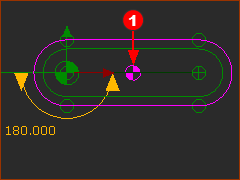Center-of-Mass Symbol(1) with Forces Display ON

 1.Mechanism-Editor: Edit the Base-Part 2.Part-Editor: Geometry toolbar > Add a Line, Add Dimension | drag to add the Line, dimensions to locate the line and define its length. 3.Part-Editor : Constraints toolbar > Add Horizontal to make the Line horizontal. Close the Part-Editor 4.Mechanism-Editor : Kinematic elements toolbar > Add Part | Drag to add the Part 5.Mechanism-Editor : Kinematics-elements toolbar > Add Pin-Joint | Click the start-Point of the Part, and an end-Point on the Line in the Base-Part. 6.Mechanism-Editor : Kinematic FB toolbar > Add Motion-Dimension FB | Click the Pin-Joint, the Lines in the Base-Part and the Part,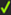the Command-Manager.

STEP 2: Add the Profile and specify its shape

 1.Edit the Rocker Part 2.Part-Editor : Geometry toolbar > Add Lines and Add Arcs (40mm Radius) | Do the Hover+Drag technique to merge the end-Points of each sketch-element (see Tutorial 3) 3.Part-Editor: Geometry toolbar > Add Coincident Constraints | to move the center-Point of each Arc to the start-Point and end-Point of the CAD-Line along the Rocker 4.Part-Editor: Geometry-Editor | Edit the length of the CAD-Line to equal 200mm. Exit the Part-Editor. 5.Mechanism-Editor: Solids menu (or toolbar) > Add Profile | Click the sketch-loop, Click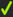in the Command-Manager. 6.Mechanism-Editor : SHIFT-CLICK the Profile 7.Selection-Window: Right-click the Extrusion element, Click Edit element to open the Extrusion dialog-box. 8.Extrusion dialog-box >  Mass-Properties : Edit the Density = 4756kg/m3 with the Extrusion Depth =10mm to make the Mass of the Extrusion = 1kg * 9.Close the Extrusion dialog-box.

* From the shape of the Extrusion, MechDesigner calculates its Mass(kg), its Moment-of-Inertia(kg.m2), and its center-of-mass.

In this case, because the sketch-loop is symmetrical and equal to the half the length of the Part - center-of-mass is 100mm from the Start-Point of the CAD-Line in the Part.

The symbol for the center-of-mass is the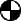- see image above#### Calculate and Display the Force Vectors

 There are two icons in the Force toolbar: Calculate and Display Force Vectors.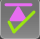1.Mechanism-Editor: Click Force toolbar > Calculate Forces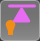1.Mechanism-Editor: Click Force toolbar > Display Force Vectors When a minimum of one Part has mass, MechDesigner will display Force Vectors. The Force-Vectors give the direction and magnitude of each Force. Notes: If necessary, increase or decrease the length of the Force Vectors. If necessary, change the colours of the Force-Vectors with the Configure-Power-Source. See Force and Torque Vector Scale buttons.

#### Analyze the ForcesForce Analysis - Information:

 •Action / Reaction force-vectors at each joint. •Moment at the Pin-Joint, which is equivalent to the Load-Torque or Application-Torque that an idealized Power Source must provide to the Rocker. •Motor symbol (in black) at the Pin-Joint. The Motor symbol identifies the location of the Power Source. •Part-Outlines changes its color. The Part-Outline and the Force-Vector that act on a Part become the same color. There are two Parts in the model - the Base-Part and the Rocker. Thus, there two(2) Force-Vector colors.Move your mouse-pointer to the Part-Outline of the Rocker.

You will see that the Force-Vectors that ACT ON the Rocker are Red

Vertical Forces (N) acting on the Rocker - upwards is +ve

 ∑FV=0 : R1(N) - 1(kg)*9.807(m/s/s) = 0;  R1 = 9.807(N) (upwards)

Moments (N.m) at the Pin-Joint - Counter-clockwise is +ve

 ∑M1=0 ; M1 - 0.020(m)*1(kg)*9.81(m/s/s) = 0 ; M1 = 0.9807(Nm)

Force Explanation of the RED Force Vectors and MomentsGravitational Vector - not shown = 1(kg)*9.807(kg/m/s/s))=9.81(N)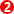Vertical Force - 9.81N acts upwards. The reaction force from the Base-Part that acts on the Rocker. The Rocker would fall freely if the Base-Part did not react to support the Rocker with the vertical Force.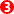Counter-Clockwise Moment of 0.98Nm. The Rocker would rotate freely clockwise if the Base-Part did not resist the Rocker with this Torque.Now move your mouse-pointer to the vertical Force-Vector that acts on the Base-Part. All vectors that ACT ON the Base-Part turn Red.

There is:Vertical Force 9.81N downwards, that acts on the Base-Part. This is the Gravitational Force that acts-on the Base-Part.Clockwise Moment (Torque) of 0.98Nm that acts on the Base-Part.Make the Rocker Vertical: Edit the Base-Value in the Motion-Dimension FB to +90º, or 270º

My mouse-pointer is above the Part-Outline of the Rocker - the Part-Outline and the Force-Vector are Red.

Vertical Force = 9.807N.

It is the equal to as when the Rocker is Horizontal. This is not a surprise. The Part has the same mass and it is the only joint through which the force can act.

Torque Moment = 0Nm.

The Center-of-Mass of the Rocker above the Pin-Joint. Therefore, a Torque is not necessary to hold the Rocker.